On Application of Development of Test Statistic for Testing Unequal Group Variances | OMICS International
Journal of Applied & Computational Mathematics

# On Application of Development of Test Statistic for Testing Unequal Group Variances

Department of Statistics, Federal University of Technology Akure, Akure, Nigeria

*Corresponding Author:
Joseph Ayodele Kupolusi
Federal University of Technology Akure
Akure, Nigeria
Tel:
+234 34 243 744
E-mail: [email protected]

Received Date: June 01, 2017; Accepted Date: July 31, 2017; Published Date: August 07, 2017

Citation: Kupolusi JA, Adebola FB (2017) On Application of Development of Test Statistic for Testing Unequal Group Variances. J Appl Computat Math 6: 359. doi: 10.4172/2168-9679.1000359

Copyright: © 2017 Kupolusi JA, et al. This is an open-access article distributed under the terms of the Creative Commons Attribution License, which permits unrestricted use, distribution, and reproduction in any medium, provided the original author and source are credited.

Visit for more related articles at Journal of Applied & Computational Mathematics

#### Abstract

In this paper, an already proposed test-statistic for testing equality of means under unequal population variances is applied. When the group variances differ, using pooled sample variance will give an inappropriate result as a single value for the variances. This kind of problem in statistics is commonly referred to as Brehen-Fisher problem in the k-sample location problems. A proposed unbiased sample harmonic mean of variances 2 HS was examined and found useful for unequal variances which have received a considerable attention in the area of medical and biological sciences. Little or nothing has been achieved in social sciences that form a major part of this work. Data from the six geopolitical zones on road crashes in Nigeria from the year 2004 to 2013 was used to ascertain the consistency of the result with the literature which was found useful and relevant for the proposed developed test statistic. It was observed that using this proposed test statistic, the number of road crashes was significant in some geopolitical zones in Nigeria which was ordinarily latent to pool sample variance.

#### Keywords

Statistic; Variances; Harmonic variances; Unequal population variances

#### Introduction

The conventional test statistic in ANOVA for testing equality of g population means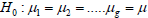against non-directional alternative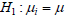for at least one i, i=1, 2, …, g, is not appropriate under the homogeneity of the variances. Instead, we might be tempted to run all possible pair wise comparisons of the population means. If we assume that all the g distributions are approximately normal with means given by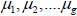and a common variance σ2 [1-5], we need to run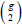t- test for comparing all pairs of means.

Obviously, this test procedure may be too tedious and time consuming. Besides, a more important but less apparent disadvantage of running multiple t-tests to compare means as stated above is that the probability of falsely rejecting at least one of hypothesis increases as the number of t-test increases (Ott, 1984). This was the origin of the Bonferroni multiple comparison procedure, (Neter and Wasserman, 1974). One difficulty with discussing the Brehrens–Fisher problem and the proposed solutions is that there are various different interpretations of what is meant by the “the Behrens–Fisher Problem”. These differences involve not only what is counted as being a relevant solution [6-8], but even the basic statement of what is being considered.

Solutions to the problem have been presented that make use of either a classical or a Bayesian inference point of view and either solution would be notionally invalid judged from the other point of view. If the consideration is left to classical statistical inference only, there is possibility of seeking solutions to the inference problem that are simple to apply in a practical sense, giving preference to this simplicity over any inaccuracy in the corresponding probability statements. Where exactness of the significance levels of statistical test is required, there may be additional requirement that the procedure should make maximum use of the statistical information in the dataset.

A proposed unbiased sample harmonic mean of variances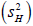was examined and found useful for unequal variances, which has received a considerable attention in the area of medical and biological sciences. Little or nothing has be achieved in social science that will form a major part of this work. Data from the six geopolitical zones on road crashes in Nigeria from the year 2004 to 2013 is considered to ascertain the consistency of the result with the literature which was found useful and relevant for the proposed developed test statistic [9-13].

Road traffic crashes have become a re-occurring phenomenon in Nigeria which constitutes a menace in modern times. Although both the developed and developing nations of the world have suffered from varying degrees of road accidents, the developing countries clearly dominates with Nigeria having the second highest rate of road traffic crashes among 193 ranked countries of the world. Deaths from reckless driving are the third leading cause of death in Nigeria. Oladepo and Brieger (1986) argued that three-quarters of all accidents on Nigerian roads involve fatalities.

#### Testing Equality of Means under Unequal Population Variances

The earliest proposed solution appeared in a paper by Behrens (1929). Fisher (1935; 1941), while acknowledging some errors in Brehens’ work, claimed to justify Behrens’ solution by the use of fiducial inference. This solution (BF test) consists of comparing the value of the sample statistics with a critical value given by an asymptotic series involving the sample variances and sample sizes. Sukhatme (1938) published tables of the 5% and 1% significance levels of the BF test. In the 1940s, W.G Cochran produced an empirical approximation based on the student’s t-table by an inspection of Sukhatme’s tables. His approximation was passed around by word of mouth and subsequently incorporated into a number of textbooks. This led Cochran in 1964 to publish an account of the accuracy of his approximation for the two-sample problem. For k=2, Cochran (1964) suggested that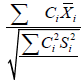could be compared to an approximate critical value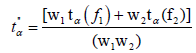Where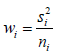In a series of papers, Welch (1938; 1947; 1951) disputed Fisher’s use of fiducial inference and rejected the claim that Brehens’ solution had been justified; he presented an approximate solution to the BF problem and published an asymptotic solution which was further studied by Aspin (1948; 1949).

Scheffe (1943) obtained a statistic for the BF problem by minimizing the length of the confidence interval for the difference of the means of two normal populations with unequal variances based on the student’s t distribution. The calculation of his confidence interval involved taking differences between sample values for subtraction was done randomly. The length of his confidence interval depended upon the arrangement of the sample values after random pairing.

Lee and garland (1975) proposed a test for the two-sample case with a critical value that depended on the sample variances, the sample sizes, and the nominal significance level. The computation of the critical value involves the solution of a nonlinear minimization problem. Abidoye et al. (2007) showed that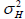harmonic mean of group variances better represents series of unequal group variances and is estimated by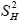. It was also shown that the sample distribution ofis approximated by the chi-square distribution.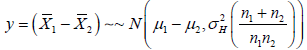(1.1)

Consequently, the test statistic for the hypothesis set in equation (1.1) is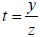(1.2)

Where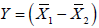(1.3)

And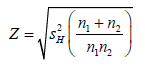(2.4)

Now p-value=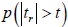(2.5)

Where is regular t-distribution and r is the appropriate degrees of freedom for the t – test. Abidoye et al. (2013a) showed that harmonic mean of group variancesbetter represents series of unequal group variances and is estimated by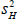. It was revealed that the sample distribution ofis approximated by the chi-square distribution.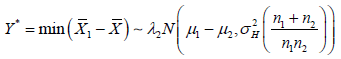(2.6)

And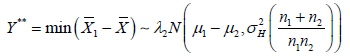(2.7)

Consequently, the test statistic for the hypotheses set in equation (2.6) is t=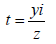(2.8)

where(2.9)

and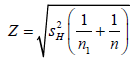(2.9.1)

Now p-value=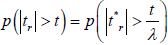(2.9.2)

Where λ can be λ1 or λ2 and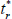is the regular t – distribution and r is the appropriate degrees of freedom for the t- test. The degree of freedom r for the harmonic mean of variances have been determined to be r=22.096+0.266(n-g) – 0.000029(n-g)2 in Abidoye et al (2013a)

Method of the proposed test statistic

We are interested in applying a developed procedure to test the hypothesis: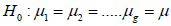against alternative,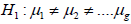, for at least one i

Where the error termThe hypothesis of equation (1) can be split into two cases that was well explained in Bonferroni test statistic, Dunnett (1964), Guptal et al. (2006) and Abidoye et al. (2007)

Define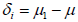(2)

Then, equation 1 can be written as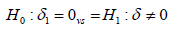(3)

Consequently the hypothesis set is written as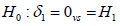case I or case II ... 4

Suppose that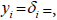the unbiased estimate of yi is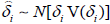Therefore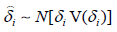Where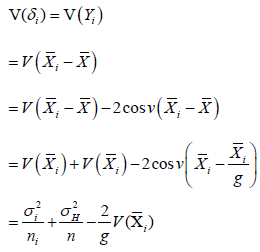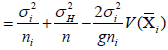Abidoye (2012), Abidoye et al. (2013c) and Abodoye et al. (2014)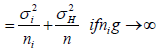Therefore,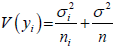(2.6)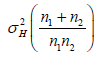Abidoye (2012)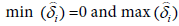Let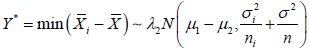(2.7)

also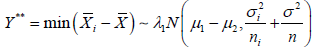(2.8)

Where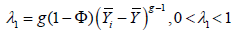Also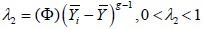obtained from the distribution of order statistic.

Distribution of harmonic variance

Abidoye et al. (2013a) explained that harmonic mean of group variancesbetter represents series of unequal group variances and is estimated by. It was shown that the sample distribution of is approximated by the chi-square distribution. His estimation is as shown in the equations below.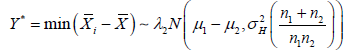(3.1)

And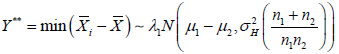(3.2)

Consequently, the test statistic for the hypotheses set in equation (3.9.1) is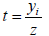(3.3)

Where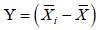(3.4)

And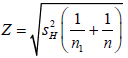(3.9.4)

Now p-value=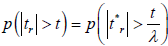(3.9.5)

Where λ can be λ1 or λ2 andis the regular t – distribution and r is the appropriate degrees of freedom for the t- test. The degree of freedom r for the harmonic mean of variances have been determined to be r=22.096+0.266(n-g) – 0.000029 (n-g)2 in Abidoye et al. (2013a)

#### Application of the Test Procedure

Secondary data on road crashes was used in this paper collected primarily by the FRSC (Federal Road Safety Commission), Nigeria. The data were grouped into six geopolitical zones (North Central, North East, North West, South East, South South and South West). Below is the table of the data for ten consecutive years covering the period of 2004 to 2013.

By the application of Levene test of equality of variances to Table 1 above, the variances differ from zone to zone which is a violation of assumed equal group variance is presented in literatures.

Years 2004 2005 2006 2007 2008 2009 2010 2011 2012 2013
North Central 1321 1534 1314 1337 2628 3109 3506 4089 3970 5110
North East 760 697 832 1002 1072 1453 1096 1605 1347 1033
North West 2158 920 1282 2189 2539 1951 2373 2826 2466 2804
South East 791 528 486 1374 1112 715 777 795 1229 1154
South South 1058 868 743 630 1380 1624 1345 1535 1664 1431
South West 8189 4526 4457 1945 2610 2002 2288 2343 2586 2051

Table 1: Geopolitical data for road crashes in Nigeria from 2004 to 2013.

Hence, we cannot use the conventional t-test statistic but that which is proposed in this paper. From the data in Tables 1 and 2 the following summary statistic were obtained.

  Levene Statistic d Response d P – Value 3.24 5 54 0.012

Table 2: Levene test for variance equality.

North Central (Zone A):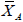=2791.8,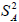=1903122.334, nA=10

North East (Zone B):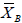=1089.7,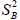=89367.57492, nB=10

North West (Zone C):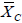=2150.8,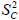=388122.3962, nC=10

South East (Zone D):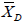=896.1,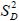=91584.97743, nD=10

South East Zone E):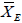=1227.8,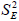=141165.7438, nE=10

SOUTH WEST (ZONE F):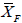= 3299.7,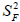=3861198.669, nF=10.

Therefore, we consider the minimum and maximum difference of means (Table 3) as shown below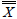2791.8 1089.7 2150.8 896.1 1227.8 3299.7 1909.32

Table 3: Table of means.

Y1=2791.8 - 1909.317 =882.483

Y2=1089.7 - 1909.317=-819.617

Y3=2150.8-1909.317=241 .4083

Y4 =8961.1-1909.317=-1013.217

Y5=1227.8–1909.317=-681.517

Y6 =3299.7-1909.317=1390.383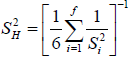Then, the minimum difference of means is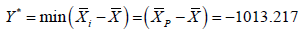From the data set above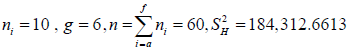The hypothesis to be tested is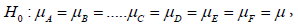Against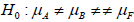for at least one i, i.e. i=A, B,…, F

And the test statistic is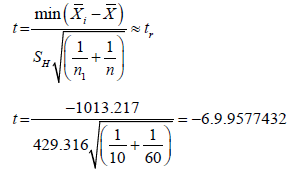Where r=22.096+0.266 (n-g)–0.000029 (n-g)2 as defined in Abidoye et.al (2013)

=22.096 + 0.266 (60-6) – 0.000029(60- 6)2

r=36.375436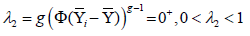Now p–value=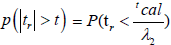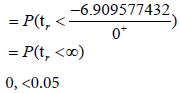In this regard, we reject H0 and conclude that average number of road crashes in all the 6 geopolitical zones are significantly different from the overall cases of road crashes at 5% level of significance. Indeed zone 6 (South West) could be the zone for which reported cases of road crash was highest and would need special attention.

Next we consider the maximum difference of means,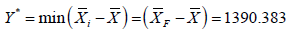The hypothesis to be tested is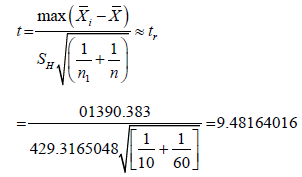Where r=36.375436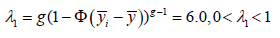Now p–value=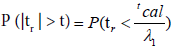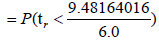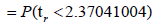= 0.011593

< 0.05

This led to the rejection of and conclusion that the mean of road crashes in all the 6 geopolitical zones are not the same at 5% level of significance.

#### Conclusion

We have applied a developed test statistic by Abidoye et al. (2013) for testing equality of means under unequal population variances to road crashes in the six geopolitical zones in Nigeria, the distribution of road crashes in these zones show that South West has the highest reported cases of road crashes and would need special attention. Since the sample harmonic mean of population variances follows a chi-square distribution, the modified t statistic is appropriated and eliminates the Behren–Fisher’s problem. Hence, sample harmonic mean of population variance is preferred to pooled sample variances of k- sample location problems.

#### References

Select your language of interest to view the total content in your interested language

### Article Usage

• Total views: 856
• [From(publication date):
September-2017 - Dec 10, 2019]
• Breakdown by view type
• HTML page views : 762Can't read the image? click here to refresh